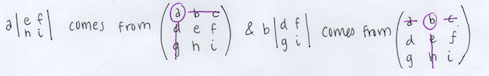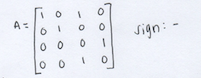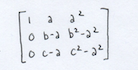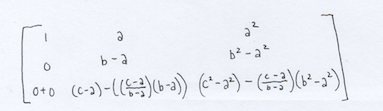# Determinants

The basic method of computing the determinant of any square size matrix

How row operations affect the determinant and how they can be used in your favor

Upper triangular form

Taken from a previous MAT 22A Linear Algebra course is this practice problem:We will go through this practice problem and I will explain how the concepts above tie in here. Let’s begin with part a.

## Computing Determinants

The formula to compute the determinant of a 2×2 matrix is as follows:Now we see how this can be used to figure out the determinant of a 3×3 matrix:The pattern seen above is that we look at the first row of matrix A, in this case (a b c), and multiply each entry of this row to the determinant of the 2×2 matrix from A that comes from “crossing out” the row and column with that corresponding entry. So for example,From there we just add and subtract the results, making sure to alternate the signs between each term.

Now we can easily work out the first part of part a above:For the second part of part a, this will be much easier after we visit row operations and upper triangular form.

## Row Operations

For any square matrix, you do not have to use the first row as the entries multiplied to the determinants. Say another row or column has more zeros than the first row, then you should use this row or column to minimize the work you need to do to find more determinants. All you need to remember is which terms to add and which terms to subtract. Here is a pattern to remember:Here are some row operation tricks you can use, but keep in mind this may change the determinant:

Switching two rows changes the sign of the determinant

Adding a multiple of a row to another row does NOT change the determinant (this can be very useful)

Multiplying a number N to a row of a matrix multiplies the determinant by the same number

## Upper Triangular Form

Upper triangular form is when we have zeros in every entry below the diagonal of the matrix. For example:

3 3 4 5

0 2 0 8

0 0 6 7

0 0 0 9

Upper triangular form allows us to just multiply the entries along the diagonal to find the determinant.

Now let’s take a look back at the second part of part a.From here, we want to use row operations to get upper triangular form. So first we swap rows 1 and 2, inversing the sign of the determinant.Now we add (-1)*row 2 to row 3 in order to get all zeros below the 1 in the second column. Note this does not change the sign of the determinant.We are very close to upper triangular form now. We just need to switch rows 3 and 4, inversing the sign of the determinant again.Finally, we have upper triangular form! Now we just multiply the entries along the diagonal to get our answer, which is 1.

We can also easily compute the third part of part a.Here, we used the first column instead of the first row because this allowed us to do the minimal amount of work to solve this problem!

Moving onto part b.Here we need to use row operations again to get upper triangular form and make this problem much easier than trying to find the determinant from the beginning.

First we want to add (-1)*row 1 to row 2 and row 3. We do this to see the (b-a) and (c-a) terms forming.Now we get upper triangular form by adding (-1)*((c-a)/(b-a))*row 2 to row 3. We found out what to multiply to row 3 by taking the term above the term we want to make 0 (in this case we want to make (c-a) 0 in row 3), and dividing (c-a) by (b-a) to make (c-a) 0 once we multiply it to row 2.Which then becomes:We now have upper triangular form, so we multiply along the diagonal to get the determinant.Finally, we have part 3 of the practice problem.Since the first edge started from (0,0,0), this allowed us to not have to find the distance between the starting point and the rest of the edges.Note: remember the volume of the box is the determinant.## Alannah Woodward

December 10, 2019

View Profile

#### Get in Touch

Thanks! We'll reach out shortly.